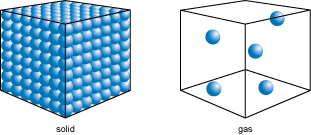An introduction to exoplanets

Start this free course now. Just create an account and sign in. Enrol and complete the course for a free statement of participation or digital badge if available.

Free course

# 4.4  Mass and density

What is mass? Mass is simply how much matter something contains. So now, think about the masses of the planets in the Solar System.

You have seen that Jupiter’s volume is more than 1000 times Earth’s volume. If, instead of being planets, Jupiter and Earth were both enormous containers, then Jupiter could hold more than 1000 times as much water as Earth. This might make you think that Jupiter is more than 1000 times as massive as Earth – but, as it happens, that isn’t the case.

Jupiter has a mass of about 1900 × 1024 kg. By 1024 we mean 1 followed by 24 zeros, so the mass of Jupiter is 1900 000 000 000 000 000 000 000 000 kg. That’s a lot of stuff! To avoid this unwieldy number, when discussing the masses of giant planets, astronomers use the mass of Jupiter, MJ, as the unit. Similarly, for terrestrial planets, the usual unit of mass is the mass of the Earth, which is given the symbol ME (or M).

Earth has a mass of about 6 × 1024 kg. So, while Jupiter is much more massive than Earth, it’s not by as much as you might have expected – a factor of around 300 rather than over 1000.

This is because the material that Jupiter is made of is less tightly packed than the material Earth is made of – Jupiter is less dense. Density is a measure of how much ‘stuff’ there is within a space of a given size. Remember that Jupiter is mostly made of hydrogen gas, whereas Earth is mostly made of rock. That means that there is less matter per unit volume in Jupiter than there is in the same volume on Earth (Figure 10).Figure 10  Relative densities of gases and solids. Particles in a solid are much closer together than particles in a gas. It’s possible to fit more matter into the same space if it is in solid form.
EXO_1